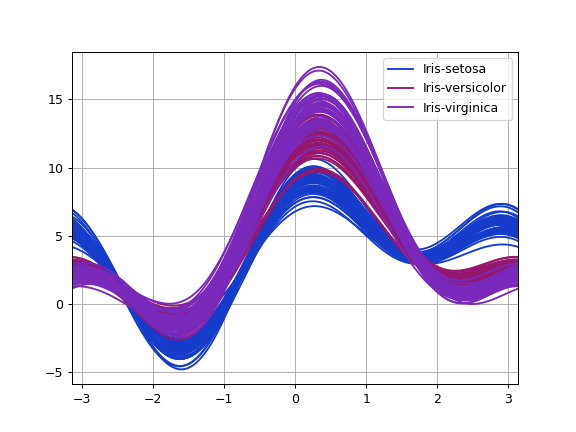# pandas.plotting.andrews_curves¶

pandas.plotting.andrews_curves(frame, class_column, ax=None, samples=200, color=None, colormap=None, **kwargs)[source]

Generate a matplotlib plot of Andrews curves, for visualising clusters of multivariate data.

Andrews curves have the functional form:

f(t) = x_1/sqrt(2) + x_2 sin(t) + x_3 cos(t) +

x_4 sin(2t) + x_5 cos(2t) + …

Where x coefficients correspond to the values of each dimension and t is linearly spaced between -pi and +pi. Each row of frame then corresponds to a single curve.

Parameters
frameDataFrame

Data to be plotted, preferably normalized to (0.0, 1.0).

class_columnName of the column containing class names
axmatplotlib axes object, default None
samplesNumber of points to plot in each curve
colorlist or tuple, optional

Colors to use for the different classes.

colormapstr or matplotlib colormap object, default None

Colormap to select colors from. If string, load colormap with that name from matplotlib.

**kwargs

Options to pass to matplotlib plotting method.

Returns
class:matplotlip.axis.Axes

Examples

```>>> df = pd.read_csv(
...     'https://raw.github.com/pandas-dev/'
...     'pandas/master/pandas/tests/io/data/csv/iris.csv'
... )
>>> pd.plotting.andrews_curves(df, 'Name')
```